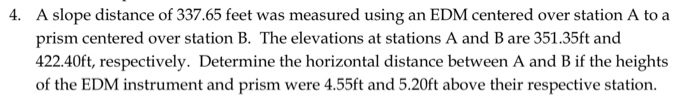# Question A slope distance of 337.65 feet was measured using an EDM centered over station A to a prism centered over station B. The elevations at stations A and B are 351.35ft and 422.40ft, respectively. Determine the horizontal distance between A and B if the heights of the EDM instrument and prism were 4.55ft and 5.20ft above their respective station 4.M2JZTJ The Asker · Civil EngineeringTranscribed Image Text: A slope distance of 337.65 feet was measured using an EDM centered over station A to a prism centered over station B. The elevations at stations A and B are 351.35ft and 422.40ft, respectively. Determine the horizontal distance between A and B if the heights of the EDM instrument and prism were 4.55ft and 5.20ft above their respective station 4.
More
Transcribed Image Text: A slope distance of 337.65 feet was measured using an EDM centered over station A to a prism centered over station B. The elevations at stations A and B are 351.35ft and 422.40ft, respectively. Determine the horizontal distance between A and B if the heights of the EDM instrument and prism were 4.55ft and 5.20ft above their respective station 4.